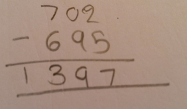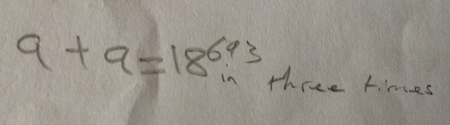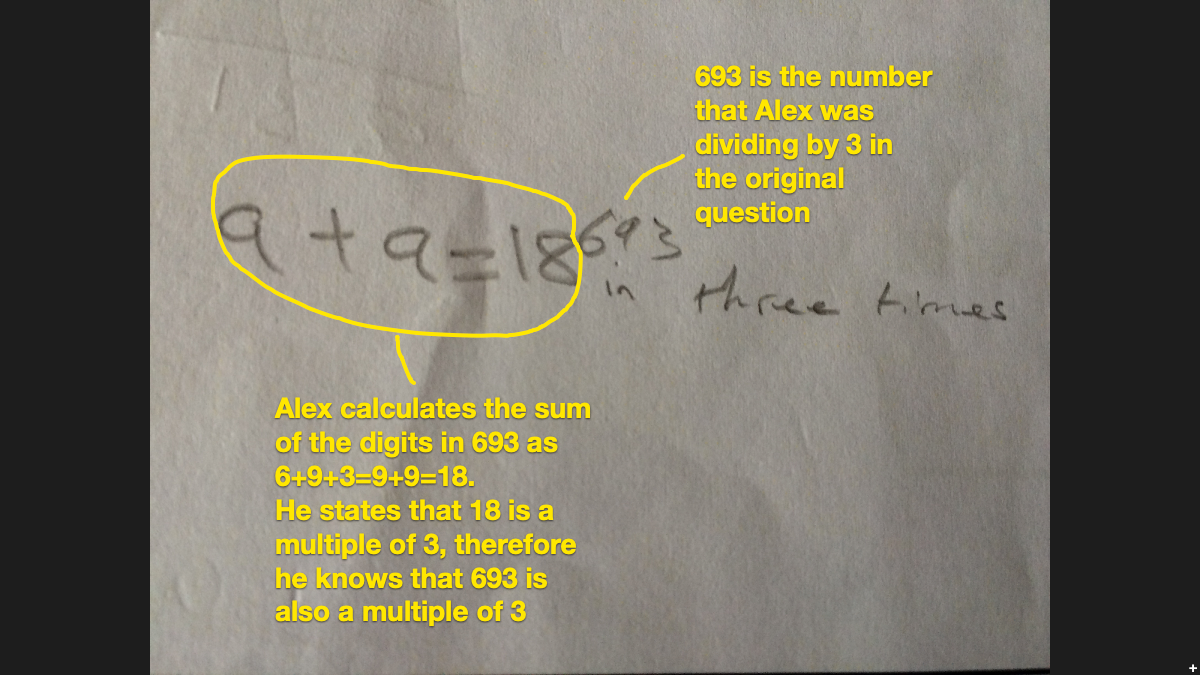# Let's Get Flexible!

##### Age 5 to 11

Published 2020

At NRICH we believe that mathematics should be an adventure, whether working through a problem to find solutions in creative ways, or taking time to explore connections between mathematical ideas. At primary level, where our curriculum is often dominated by the expectation that learners should fluently perform formal algorithms, we still need to ensure that our learners experience opportunities to be adventurous and work flexibly:

The reason that one problem can be solved in multiple ways is that mathematics does not consist of isolated rules but connected ideas. Being able to and tending to solve a problem in more than one way, therefore, reveals the ability and the predilection to make connections between and among mathematical areas and topics. (Ma, 1999)

One way to encourage a sense of adventure, and develop algorithmic fluency, is by comparing different ways to perform a calculation. Picture Your Method is an example of an NRICH task which focuses entirely on answering a calculation in more than one way, and it invites learners to consider how each method might be represented pictorially.

Take a moment to consider how many ways you could calculate 702 - 695. How might you expect an 8-year-old to complete the calculation? How about an 11-year-old? Recent research indicated that most 11-year-olds chose the formal subtraction algorithm, but counting-up was a significantly more accurate strategy (Lord, 2019). One common mistake using the formal algorithm was totalling the numbers rather than finding their difference. Here is Kate's calculation:Being fluent goes beyond knowing how to perform a formal algorithm. Mathematical fluency requires our learners to recognise occasions when they should not apply a formal algorithm; small differences, easily rounded numbers and near doubles do not necessarily require written methods. The task Subtraction Slip deliberately includes a mistake, which gives learners the opportunity to think carefully about their choice of method. We know that learners can be significantly more accurate when they choose the most appropriate strategy based on the merits of each individual calculation. The two problems Arranging Additions and Sorting Subtractions, and Compare the Calculations offer a chance for children to do just that.

Learners can improve their accuracy rates even further if they check their answers using a different calculation strategy. For example, here are Alex's jottings:Alex explained that he quickly checked his answer for the calculation 693 Ã· 3 by applying his knowledge of divisibility rules. He knew that if the sum of the digits of a number is divisible by three, then the number is divisible by 3. Since the sum of the digits for 693 totals 18, which is divisible by 3, Alex knew that 693 is divisible by 3.This flexible approach offers our learners the opportunity to experience more decision-making in their number work, enhancing their mathematical curiosity and sense of adventure. It has been likened to owning a mathematical toolbox, filled with different calculation strategies' merits, which we can model with our learners to ensure that they develop a willingness to use them.

In our feature, A Flexible Approach to Calculation, you can find a range of tasks (some of which are mentioned above) to support you in encouraging learners to make, and justify, decisions about the method they use to solve a calculation.

References

Lord, E. L. (2019). Calculation fluency: A mixed methods study in English Y6 primary classrooms (Doctoral dissertation, University of Cambridge).

Ma, L. (1999). Knowing and teaching elementary mathematics: Teachers' understanding of fundamental mathematics in China and the United States. Mahwah, NJ: Lawrence Erlbaum Associates, Inc.